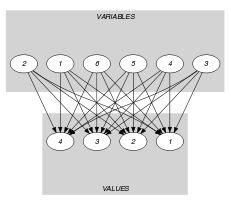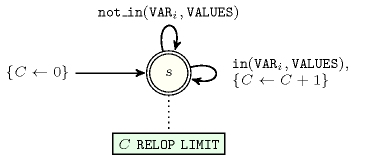## 5.92. counts

Origin

Derived from $\mathrm{𝚌𝚘𝚞𝚗𝚝}$.

Constraint

$\mathrm{𝚌𝚘𝚞𝚗𝚝𝚜}\left(\mathrm{𝚅𝙰𝙻𝚄𝙴𝚂},\mathrm{𝚅𝙰𝚁𝙸𝙰𝙱𝙻𝙴𝚂},\mathrm{𝚁𝙴𝙻𝙾𝙿},\mathrm{𝙻𝙸𝙼𝙸𝚃}\right)$

Arguments
 $\mathrm{𝚅𝙰𝙻𝚄𝙴𝚂}$ $\mathrm{𝚌𝚘𝚕𝚕𝚎𝚌𝚝𝚒𝚘𝚗}\left(\mathrm{𝚟𝚊𝚕}-\mathrm{𝚒𝚗𝚝}\right)$ $\mathrm{𝚅𝙰𝚁𝙸𝙰𝙱𝙻𝙴𝚂}$ $\mathrm{𝚌𝚘𝚕𝚕𝚎𝚌𝚝𝚒𝚘𝚗}\left(\mathrm{𝚟𝚊𝚛}-\mathrm{𝚍𝚟𝚊𝚛}\right)$ $\mathrm{𝚁𝙴𝙻𝙾𝙿}$ $\mathrm{𝚊𝚝𝚘𝚖}$ $\mathrm{𝙻𝙸𝙼𝙸𝚃}$ $\mathrm{𝚍𝚟𝚊𝚛}$
Restrictions
 $\mathrm{𝚛𝚎𝚚𝚞𝚒𝚛𝚎𝚍}$$\left(\mathrm{𝚅𝙰𝙻𝚄𝙴𝚂},\mathrm{𝚟𝚊𝚕}\right)$ $\mathrm{𝚍𝚒𝚜𝚝𝚒𝚗𝚌𝚝}$$\left(\mathrm{𝚅𝙰𝙻𝚄𝙴𝚂},\mathrm{𝚟𝚊𝚕}\right)$ $\mathrm{𝚛𝚎𝚚𝚞𝚒𝚛𝚎𝚍}$$\left(\mathrm{𝚅𝙰𝚁𝙸𝙰𝙱𝙻𝙴𝚂},\mathrm{𝚟𝚊𝚛}\right)$ $\mathrm{𝚁𝙴𝙻𝙾𝙿}\in \left[=,\ne ,<,\ge ,>,\le \right]$
Purpose

Let $N$ be the number of variables of the $\mathrm{𝚅𝙰𝚁𝙸𝙰𝙱𝙻𝙴𝚂}$ collection assigned to a value of the $\mathrm{𝚅𝙰𝙻𝚄𝙴𝚂}$ collection. Enforce condition $N\mathrm{𝚁𝙴𝙻𝙾𝙿}\mathrm{𝙻𝙸𝙼𝙸𝚃}$ to hold.

Example
$\left(〈1,3,4,9〉,〈4,5,5,4,1,5〉,=,3\right)$

Values 1, 3, 4 and 9 of the $\mathrm{𝚅𝙰𝙻𝚄𝙴𝚂}$ collection are assigned to 3 items of the $\mathrm{𝚅𝙰𝚁𝙸𝙰𝙱𝙻𝙴𝚂}=〈4,5,5,4,1,5〉$ collection. The $\mathrm{𝚌𝚘𝚞𝚗𝚝𝚜}$ constraint holds since this number is in fact equal ($\mathrm{𝚁𝙴𝙻𝙾𝙿}$ is set to $=$) to the last argument of the $\mathrm{𝚌𝚘𝚞𝚗𝚝𝚜}$ constraint.

Typical
 $|\mathrm{𝚅𝙰𝙻𝚄𝙴𝚂}|>1$ $|\mathrm{𝚅𝙰𝚁𝙸𝙰𝙱𝙻𝙴𝚂}|>1$ $\mathrm{𝚛𝚊𝚗𝚐𝚎}$$\left(\mathrm{𝚅𝙰𝚁𝙸𝙰𝙱𝙻𝙴𝚂}.\mathrm{𝚟𝚊𝚛}\right)>1$ $|\mathrm{𝚅𝙰𝚁𝙸𝙰𝙱𝙻𝙴𝚂}|>|\mathrm{𝚅𝙰𝙻𝚄𝙴𝚂}|$ $\mathrm{𝚁𝙴𝙻𝙾𝙿}\in \left[=,<,\ge ,>,\le \right]$ $\mathrm{𝙻𝙸𝙼𝙸𝚃}>0$ $\mathrm{𝙻𝙸𝙼𝙸𝚃}<|\mathrm{𝚅𝙰𝚁𝙸𝙰𝙱𝙻𝙴𝚂}|$
Symmetries
• Items of $\mathrm{𝚅𝙰𝙻𝚄𝙴𝚂}$ are permutable.

• Items of $\mathrm{𝚅𝙰𝚁𝙸𝙰𝙱𝙻𝙴𝚂}$ are permutable.

• An occurrence of a value of $\mathrm{𝚅𝙰𝚁𝙸𝙰𝙱𝙻𝙴𝚂}.\mathrm{𝚟𝚊𝚛}$ that belongs to $\mathrm{𝚅𝙰𝙻𝚄𝙴𝚂}.\mathrm{𝚟𝚊𝚕}$ (resp. does not belong to $\mathrm{𝚅𝙰𝙻𝚄𝙴𝚂}.\mathrm{𝚟𝚊𝚕}$) can be replaced by any other value in $\mathrm{𝚅𝙰𝙻𝚄𝙴𝚂}.\mathrm{𝚟𝚊𝚕}$ (resp. not in $\mathrm{𝚅𝙰𝙻𝚄𝙴𝚂}.\mathrm{𝚟𝚊𝚕}$).

Arg. properties
• Contractible wrt. $\mathrm{𝚅𝙰𝚁𝙸𝙰𝙱𝙻𝙴𝚂}$ when $\mathrm{𝚁𝙴𝙻𝙾𝙿}\in \left[<,\le \right]$.

• Extensible wrt. $\mathrm{𝚅𝙰𝚁𝙸𝙰𝙱𝙻𝙴𝚂}$ when $\mathrm{𝚁𝙴𝙻𝙾𝙿}\in \left[\ge ,>\right]$.

• Aggregate: $\mathrm{𝚅𝙰𝙻𝚄𝙴𝚂}\left(\mathrm{𝚜𝚞𝚗𝚒𝚘𝚗}\right)$, $\mathrm{𝚅𝙰𝚁𝙸𝙰𝙱𝙻𝙴𝚂}\left(\mathrm{𝚞𝚗𝚒𝚘𝚗}\right)$, $\mathrm{𝚁𝙴𝙻𝙾𝙿}\left(\mathrm{𝚒𝚍}\right)$, $\mathrm{𝙻𝙸𝙼𝙸𝚃}\left(+\right)$ when $\mathrm{𝚁𝙴𝙻𝙾𝙿}\in \left[<,\le ,\ge ,>\right]$.

Usage

Used in the Constraint(s) on sets slot for defining some constraints like $\mathrm{𝚊𝚜𝚜𝚒𝚐𝚗}_\mathrm{𝚊𝚗𝚍}_\mathrm{𝚌𝚘𝚞𝚗𝚝𝚜}$.

Reformulation

The $\mathrm{𝚌𝚘𝚞𝚗𝚝}$$\left(\mathrm{𝚅𝙰𝙻𝚄𝙴𝚂},\mathrm{𝚅𝙰𝚁𝙸𝙰𝙱𝙻𝙴𝚂},\mathrm{𝚁𝙴𝙻𝙾𝙿},\mathrm{𝙻𝙸𝙼𝙸𝚃}\right)$ constraint can be expressed in term of the conjunction $\mathrm{𝚊𝚖𝚘𝚗𝚐}$$\left(N,\mathrm{𝚅𝙰𝚁𝙸𝙰𝙱𝙻𝙴𝚂},\mathrm{𝚅𝙰𝙻𝚄𝙴𝚂}\right)\wedge N\mathrm{𝚁𝙴𝙻𝙾𝙿}\mathrm{𝙻𝙸𝙼𝙸𝚃}$.

Systems

count in Gecode.

Used in

specialisation: $\mathrm{𝚌𝚘𝚞𝚗𝚝}$ ($\mathrm{𝚟𝚊𝚛𝚒𝚊𝚋𝚕𝚎}\in \mathrm{𝚅𝙰𝙻𝚄𝙴𝚂}$ replaced by $\mathrm{𝚟𝚊𝚛𝚒𝚊𝚋𝚕𝚎}$=$\mathrm{𝚅𝙰𝙻𝚄𝙴}$).

Keywords
Arc input(s)

$\mathrm{𝚅𝙰𝚁𝙸𝙰𝙱𝙻𝙴𝚂}$ $\mathrm{𝚅𝙰𝙻𝚄𝙴𝚂}$

Arc generator
$\mathrm{𝑃𝑅𝑂𝐷𝑈𝐶𝑇}$$↦\mathrm{𝚌𝚘𝚕𝚕𝚎𝚌𝚝𝚒𝚘𝚗}\left(\mathrm{𝚟𝚊𝚛𝚒𝚊𝚋𝚕𝚎𝚜},\mathrm{𝚟𝚊𝚕𝚞𝚎𝚜}\right)$

Arc arity
Arc constraint(s)
$\mathrm{𝚟𝚊𝚛𝚒𝚊𝚋𝚕𝚎𝚜}.\mathrm{𝚟𝚊𝚛}=\mathrm{𝚟𝚊𝚕𝚞𝚎𝚜}.\mathrm{𝚟𝚊𝚕}$
Graph property(ies)
$\mathrm{𝐍𝐀𝐑𝐂}$$\mathrm{𝚁𝙴𝙻𝙾𝙿}\mathrm{𝙻𝙸𝙼𝙸𝚃}$

Graph class
 $•$$\mathrm{𝙰𝙲𝚈𝙲𝙻𝙸𝙲}$ $•$$\mathrm{𝙱𝙸𝙿𝙰𝚁𝚃𝙸𝚃𝙴}$ $•$$\mathrm{𝙽𝙾}_\mathrm{𝙻𝙾𝙾𝙿}$

Graph model

Because of the arc constraint $\mathrm{𝚟𝚊𝚛𝚒𝚊𝚋𝚕𝚎𝚜}.\mathrm{𝚟𝚊𝚛}=\mathrm{𝚟𝚊𝚕𝚞𝚎𝚜}.\mathrm{𝚟𝚊𝚕}$ and since each domain variable can take at most one value, $\mathrm{𝐍𝐀𝐑𝐂}$ is the number of variables taking a value in the $\mathrm{𝚅𝙰𝙻𝚄𝙴𝚂}$ collection.

Parts (A) and (B) of Figure 5.92.1 respectively show the initial and final graph associated with the Example slot. Since we use the $\mathrm{𝐍𝐀𝐑𝐂}$ graph property, the arcs of the final graph are stressed in bold.

##### Figure 5.92.1. Initial and final graph of the $\mathrm{𝚌𝚘𝚞𝚗𝚝𝚜}$ constraint(a) (b)
Automaton

Figure 5.92.2 depicts the automaton associated with the $\mathrm{𝚌𝚘𝚞𝚗𝚝𝚜}$ constraint. To each variable ${\mathrm{𝚅𝙰𝚁}}_{i}$ of the collection $\mathrm{𝚅𝙰𝚁𝙸𝙰𝙱𝙻𝙴𝚂}$ corresponds a 0-1 signature variable ${S}_{i}$. The following signature constraint links ${\mathrm{𝚅𝙰𝚁}}_{i}$ and ${S}_{i}$: ${\mathrm{𝚅𝙰𝚁}}_{i}\in \mathrm{𝚅𝙰𝙻𝚄𝙴𝚂}⇔{S}_{i}$.

##### Figure 5.92.2. Automaton of the $\mathrm{𝚌𝚘𝚞𝚗𝚝𝚜}$ constraint##### Figure 5.92.3. Hypergraph of the reformulation corresponding to the automaton (with one counter) of the $\mathrm{𝚌𝚘𝚞𝚗𝚝𝚜}$ constraint: since all states variables ${Q}_{0},{Q}_{1},\cdots ,{Q}_{n}$ are fixed to the unique state $s$ of the automaton, the transitions constraints share only the counter variable $C$ and the constraint network is Berge-acyclic